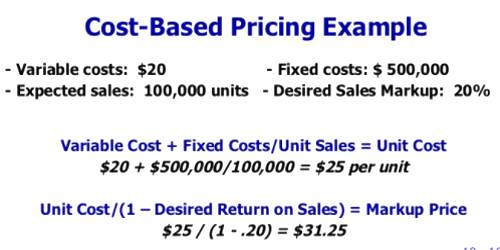Cost Based on Unit Price

Cost-based pricing involves setting prices based on the costs of producing, distributing and selling the product. Also, the company normally adds a fair rate of return to compensate for its efforts and risks. The total cost of a number of items may be calculated when the unit cost is known and the quantity that will be purchased is decided. For example, if a caterer will serve a meal for \$6.25 per person and 100 people will be served, the total cost will be \$6.25*100 or \$625

You may also need to calculate total cost based on unit cost and the number of items when you are ordering several items of the same kind.Explanation

The cost per unit is derived from the variable costs and fixed costs incurred by a production process, divided by the number of units produced. Variable costs, such as direct materials, very roughly in proportion to the number of units produced, though this cost should decline somewhat as unit volumes increase, due to greater purchasing discounts.

Within these restrictions, then, the cost per unit calculation is:

(Total fixed costs + Total variable costs) ÷ Total units produced.

The cost per unit should decline as the number of units produced increases, primarily because the total fixed costs will be spread over a larger number of units (subject to the step costing issue noted above). Thus, the cost per unit is not constant.

For example, ABC Company has total variable costs of \$50,000 and total fixed costs of \$30,000 in May, which it incurred while producing 10,000 widgets. The cost per unit is:

(\$30,000 Fixed costs + \$50,000 variable costs) ÷ 10,000 units = \$8 cost per unit

In the following month, ABC produces 5,000 units at a variable cost of \$25,000 and the same fixed cost of \$30,000. The cost per unit is:

(\$30,000 Fixed costs + \$25,000 variable costs) ÷ 5,000 units = \$11/unit.

In simple example:

For example, at XYZ Restaurant, which sells only pepperoni pizza, the variable expenses per pizza might be:

Flour- \$0.50; Yeast- \$0.05; Water- \$0.01; Cheese- \$3.00; Pepperoni- \$2.00

Total- \$5.56 per pizza

Its fixed expenses per month might be:

Labor: \$1,500; Rent: \$3,000; Insurance: \$200; Advertising: \$500; Utilities: \$450

Total: \$5,650

If company XYZ sells 10,000 pizzas, then its cost per unit would be:

Cost per unit = \$5.56 + (\$5,650/10,000) = \$6.125

When figuring your total costs, including both your fixed costs and your variable costs. Fixed costs include ones that don’t change based on the number of units you make, such as rent or property taxes. Variable costs do change based on how much you produce, such as the cost for the raw materials you use, machinery that needs to be replaced after making a certain number of units and the wages for your employees.

Information Source: